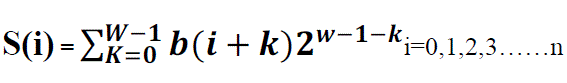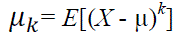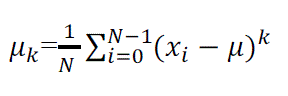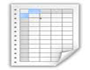ISSN ONLINE(2320-9801) PRINT (2320-9798)

All submissions of the EM system will be redirected to Online Manuscript Submission System. Authors are requested to submit articles directly to Online Manuscript Submission System of respective journal.

# A Novel Technique for Detection of CVSD Encoded Bit Stream

 Parthraj Tripathi1, Dr.K.Padma Raju2, V.Ravi Chandra3 Scientist, Defence Electronics Research Laboratory, DRDO, Hyderabad, India Principal, University College of Engineering, JNTUK, Kakinada, India Department of Electronics and Communication Engineering, University College of Engineering, JNTUK, Kakinada, India Related article at Pubmed, Scholar Google

Visit for more related articles at International Journal of Innovative Research in Computer and Communication Engineering

## Abstract

in tactical communication where the quality of signal is option for the secure transmission,Continuously Variable Slope Delta modulation(CVSD) codec is used which is very difficult to decodeif intercepted because of its adaptive nature and likewise its parameters.In communication systemswhere this codec is widely used, required a method to detect or identify the bitstream of this kind.in this paper we attempt to extend classifier algorithm to identify CVSD codec by extracting 7 different parameters for each windowsize operation from the incoming unknown bit stream and compare with the thresholds are already calculated and stored in database.operations are performed on different slides 8,10,16,24 and 32 to extract total of 35 parameters,all these parameter values are added to previous already existing library.

### INTRODUCTION

reducing the signal bandwidth without degrade in its quality is challenging part of modern communications,for this purpose many codecs are existing in real time like PCM A-law/U-law,Adpcm at different data rates 16-,24-,32-,40 kbps,where the bandwidthof signal reduced by reducing no of bits per sample without degrade in the quality of signal, but above standards are not secure in transmitting through channel. .in military communication system quality is option to the secure transmission is called tactical communication system.for this one bit per sample modulation delta modulation is evolved with simple and secure communications.but this algorithm has serious issue of slope overload distortion and granular noise because of fixed step size to overcome thisContinuously Variable Slope Delta modulation ( CVSD ) codec is designed and implemented with variable step size.

### RELATED WORK

This paper we attempt to extend the classifier algorithm. which is capable of identifying PCM ?? /A-Law, ADPCM 16-,24-,32-,40 Kbps, here we introduced CVSD codec to the existing library by extracting the novel parameters from CVSD encoded bit streams by using novel technique called 'SWOB'(sliding window on bit stream) technique, amalgamated with seven parameters namely AC (auto correlation), SOM (central second order moment) and BRO(binary ratio), skewness (central third order moment),central fourth order moment, central fifth order moment.
The main contribution of this paper can be summarized as follows:
Introduction about need of CVSD codec in modern communications.
Introduction of the novel technique with detailed explanation of the classifier algorithm.
briefdescription about the parameters used in the classifier.
Experimental results are tabulated.

### CLASSIFIER ALGORITHM

It is an attempt to extend the capability of the existing classifier algorithm .by adding CVSD codec parameters to the library.the bit stream received through the speech discriminator divided into the different 2000 bit segments. for each segment sliding window technique (SWOB) is performed which divides into 8,10,16,24,32 bit frames. First five central order moments, autocorrelation and binary ratio of each segment is calculated compared in the values in decisionbox which identifies the class of incoming signal.
Figure 2 shows the block diagram of the classifier algorithm which is capable of identifying and classifying the digital voice bit stream. By incorporating this classification algorithm in the universal decoder design, its performance can be improved when it analyses the large number of the unclassified signal.

### CLASSIFICATION TECHNIQUE AND PARAMETERS

#### SWOB' Technique:

This technique is novel technique and it is applied on the received bit stream and it will generate different slides of different sizes 8,10,16,24 and 32 from the group of bits. The mathematical representation of sliding window technique is represented asWhere, b(i) is received bit stream, S(i) is the decimal values obtained from the window technique, w is the sliding window size and n is the number of received bits.
The sliding window technique process is shown in figure 3 and as follows: First, choose the size of sliding window and calculate the decimal value from the bits present in the window. Second, slide the window by one bit and calculate its decimal (byte) equivalent and this process continue for remaining all bits in the received bit stream. In our case of analysis, the size of sliding window was taken as eight. After calculating the decimal equivalent values from the sliding window technique, auto correlation sequence and first five central order moments are calculated on these decimal values in order to classify the digital voice bit stream.

#### mean:

It calculates the average of all the sequence of slides of the incoming bit stream .

#### Autocorrelation Sequence:

The autocorrelation represents degree of similarity between a given time series and a lagged version of itself over successive time intervals . The mathematical expression of the auto correlation sequence of signal x(n) is represented as follows , :Where,* represents the complex conjugate, k is the lag and R(k) is the auto correlation sequence of the signal. Here, we are not performing the complex conjugate computation. So the ACS equation is rewritten asWhere s(i) is the real values generated from the sliding window technique, N is the segment length, and k is the lag tocompute. In order to reduce the multiplications, we use real ACS rather than complex valued one.

#### Central moments:

First five Central order moments are calculated to the different slides of incoming bitstream measures the width of a set of points in one dimension and measures the shape of a cloud of points for higher dimensions . The mathematical representation of central order moment of s(i) is given as ,:µk is the kth central order moment.BRO 'Binary Ratio:
Since there is variation in the lower level and higher level ratio for different codecs.so BRO is used as a parameter in classifying the digital voice bit stream.BRO is calculated on the each 2000 segments of incoming bit stream..
If b(i)=0; then ZR=ZR+1.
If b(i)=0; then ZR=ZR+1
Then the binary ratio (BRO) is given as BRO=ZR/OR.
Where, ZR is the number of lower levels in digital bit stream. OR is the number of higher levels in digital bit stream.

### EXPERIMENTAL RESULTS

Prior to testing the algorithm, a large body of test signals was generated. The voice signals are recorded using microphone and sampled at 8000 samples per second. These samples are stored in int16 format and encoded using CVSD encoding algorithms. All the testing signals are processed using classifier algorithm it is observed that CVSD class signal is discriminated.
Based up on the results obtained after analyzing large set of test body the thresholds are obtained for each class are as shown in table 1. These thresholds values of each class are stored in the decision box and based up on these thresholds we can detect the captured bit stream as CVSD.c
Based up on the results obtained after analyzing large set of test body the thresholds are obtained for each class are as shown in table 1. These thresholds values of each class are stored in the decision box andbased up on these thresholds we can detect the captured bit stream as CVSD.

### CONCLUSION AND FUTURE WORK

In this paper,we described a digital voice signal classifier that accurately identifies the digital voice bit stream.this classifier is capable of identifying CVSD at different data rates.it will classify the digital voice bit streamand that too when the bit stream are not synchronized i.e. start bit is not identified,for a signal captured, the classifier algorithm calculates all 7 parameters namely binary ratio, auto correlation, mean,variance,third order moment,fourth order moment, fifth order moment, of the received signal followed by comparision of threshold values stored in the decision box,it classifies any given bit stream.these threshold values are added to the already existing library.
This classifier algorithm is more reliable and less complex than the traditional and widely used techniques for un known bitstream identification.the future work can be done on classifying other signal types namely image,video etc.

### Tables at a glanceTable 1

### Figures at a glanceFigure 3 Figure 4